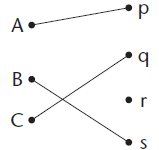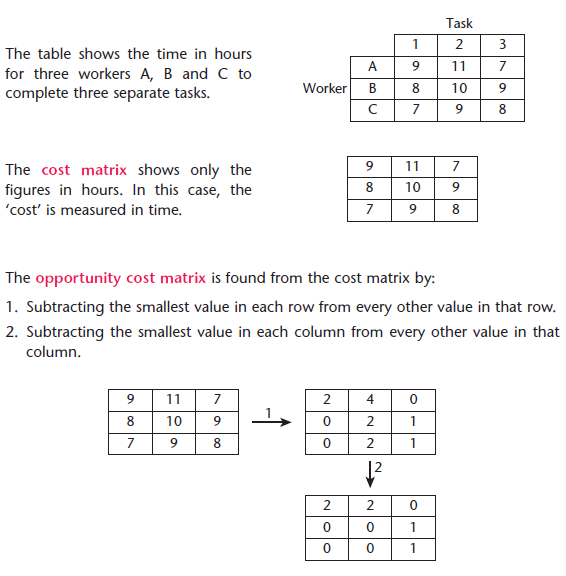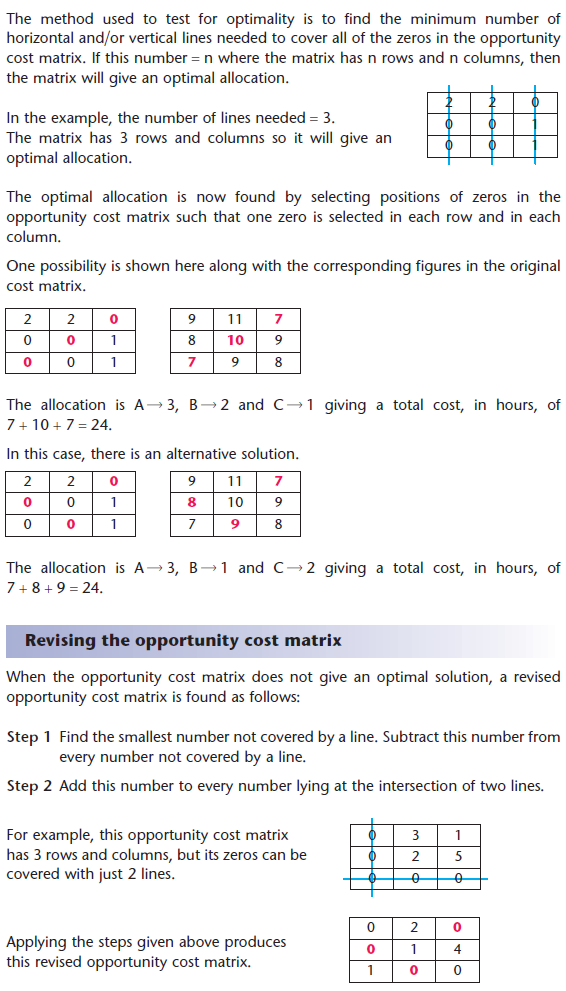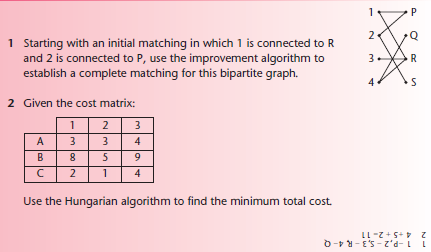## Title

Matching and allocation
Quick revise

After studying this section you should be able to:

• use bipartite graphs to model matchings
• understand the conditions for matchings to be maximal or complete
• apply the maximum matching algorithm

Matchings and graphs

A bipartite graph is a graph in which the vertices are divided into two sets such that no pair of vertices in the same set is connected by an edge. In this case, the two sets are {A, B, C} and {p, q, r, s}.Some vertices in a bipartite graph may not be connected to another vertex.

A matching between two sets may be represented by a bipartite graph in which there is at most one edge connecting a pair of vertices.

A maximal matching is a matching which has the maximum number of edges. This occurs when every vertex in one of the sets is connected to a vertex in the other set. The bipartite graph shown above represents a maximal matching.

A complete matching is a matching in which every vertex is connected to another vertex. This can only occur when the two sets contain the same number of vertices.

The matching improvement algorithm

Figure 1 is a bipartite graph showing the possible connections between two sets. It does not represent a matching because some vertices have more than one connection.

Figure 2 is a bipartite graph representing an initial matching.

An initial matching may be improved by increasing the number of connections. This is the purpose of the matching improvement algorithm.

• Step 1 In the initial matching, start from a vertex that is not connected and look for an alternating path to a vertex in the other set that is not connected.
• Step 2 Each edge on the alternating path, not included in the initial matching, is now included and each edge originally included is removed.
• Step 3 Repeat steps 1 and 2 using the latest matching in place of the initial matching until no further alternating paths can be found.

In an alternating path, the edges alternate between those from the bipartite graph that are not in the initial matching and those that are.

Allocation problems

In an allocation (or assignment) problem, the object is to set up a matching that will optimise a particular objective, such as minimising a cost or maximising a profit. If we regard one set of objects as agents and the other set as tasks, then the
underlying assumptions are that:

• Any agent may be assigned to any task (unlike the matching problems).
• Each agent is assigned at most one task and each task has at most one agent assigned to it.

When the number of agents is equal to the number of tasks, the problem is said to be balanced. If the number of agents exceeds the number of tasks, or vice versa, then the problem is unbalanced. The technique for solving an unbalanced problem involves introducing a dummy agent or task which then allows the solution to proceed as for the balanced case. In the solution of an unbalanced problem, a task assigned to a dummy agent isn’t actually completed and an agent assigned to a dummy task doesn’t actually have a task to carry out.

The opportunity cost matrixThe Hungarian algorithm

The Hungarian algorithm is used to find the allocation that optimises the given objective.

• Step 1 Find the opportunity cost matrix.
• Step 2 Test for optimality. If true then make the allocation and stop.
• Step 3 Revise the opportunity cost matrix and repeat step 2.Maximising allocation problems

If the objective involves maximising a quantity, such as a profit, then the first step is to subtract every value in the original matrix from a fixed value. This fixed value may be taken to be the largest value in the matrix or any value larger than this. The Hungarian algorithm is now applied in the usual way to this transformed matrix.

PROGRESS CHECKRate: